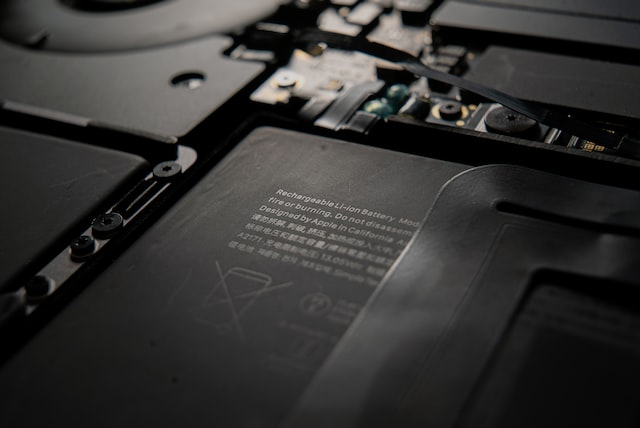# Designing a simple BMS for electric vehiclesElectric vehicles (EVs) or Battery Electric Vehicles (BEVs) are vehicles that rely completely on batteries for power. Battery cells used in vehicles are largely made from chemical compounds such as Lithium which are susceptible to temperature and pressure changes. In order to ensure battery longevity, the cells must be operated within a safe operating boundary. In our August 2022 blog, we covered the basics of a battery management system which is to ensure the state of a battery is adequately reported and regulated, and detailed why it is a crucial part of battery system design.

In this tutorial, we will learn how to design a simple battery management system in Collimator.

## Battery Management System (BMS) model overview

The battery management system design is made up of five components which we will model in Collimator as follows:

1. Vehicle load model - Simulates the charging and discharging current cycles of the car
2. BMS controller model - Simulates the monitoring of all battery parameters, including the main state machine and the State of Charge (SOC)
3. Battery Plant model - Battery plant specified to an industry standard lithium ion battery in the form of a lookup table
4. BMS voltage and current sensor
5. BMS temperature sensor

An overview of the model is shown below:

## Charging and discharging current cycles

The vehicle load demand model, shown below, emulates the charging and discharging current cycles of the vehicle. We model two different vehicle load demands: 1) a pulse with an offset and 2) a constant:

We employ an if/else logic statement to switch between the two:

## BMS controller

The primary function of a BMS is to meet the safety requirements for operating a battery under various load and environmental conditions. This ensures lower risk during the operation. Our Battery Management System is composed of 4 parts:

1. Current saturation
2. Cell current monitoring
3. Cell voltage monitoring
4. Temperature monitoring

When done well, this will allow us to increase the efficiency of the battery and prolong battery life, thus reducing the total cost of ownership of our electric vehicle.

### 1. Current Saturation

Current saturation is used to moderate the demand from the driver and ensure that the vehicle does not draw more current than is safe or good for the battery. In our case, we will limit the vehicle load current to predetermined min and max constant values 1 and -1 respectively. Below, we compare the max current (batt_current_max_A) to the min current (batt_current_min) and vehicle demand (veh_load_current_ref_A_0).

We then use a saturation block to limit the output current within the constants defined.

### 2. Cell Current Monitoring

The cell current monitoring begins with the ADC current count:

We run a digital audio conversion of the ADC count, add the offset constant, and multiply this by the resistor constant in order to get the battery current. We'll employ some fault detection using logic operators to ensure our current is within the maximum and minimum amp values.

Next, the main state machine sub-model takes the current and employs if/else statements in Python to identify the state of the battery.

if time == 0:
from enum import Enum
class State(Enum):
IDLE = 0
DISCHARGING = 1
CHARGING = 2
class Control(Enum):
Idle = 0
Discharge = 1
Charge = 2
tick = 0
state = State.IDLE

if state is State.IDLE:
if I_BATT == 0:
control = Control.Idle.value
tick = tick + 1;
elif I_BATT > 0:
control = Control.Discharge.value
state = State.DISCHARGING
tick = 0
elif I_BATT < 0:
control = Control.Charge.value
state = State.CHARGING
tick = 0
elif state is State.DISCHARGING:
if I_BATT > 0:
control = Control.Discharge.value
tick = tick + 1;
elif I_BATT == 0:
control = Control.Idle.value
state = State.IDLE
tick = 0
elif I_BATT < 0:
control = Control.Charge.value
state = State.CHARGING
tick = 0
elif state is State.CHARGING:
if I_BATT < 0:
control = Control.Charge.value
tick = tick + 1;
elif I_BATT == 0:
control = Control.Idle.value
state = State.IDLE
tick = 0
elif I_BATT > 0:
control = Control.Discharge.value
state = State.DISCHARGING
tick = 0




Lastly, our fuel gauge monitor sub-model will estimate the battery percentage and the amount of current stored in the battery.

### 3. Cell Voltage Monitoring

Similar to the cell current monitoring, the cell voltage monitoring is responsible for converting ADC voltage count in bits to voltage in volts. We will also ensure fault detection is in place to check for overvoltage and undervoltage.

If either the current or voltage is at fault, the battery as a whole will be inoperable and at fault.

### 4. Cell Temperature Monitoring

For the purpose of this simulation we are assume a constant battery temperature.

## The battery plant model

The battery plant model is implemented using physics based electrical circuit equations along with data from the manufacturer to closely model a generic Lithium-ion (Li-Ion) battery. The model calculates the internal voltage drop from internal resistance and battery current information. Then a 1D lookup table is used to estimate open circuit voltage from the SOC which is estimated using coulomb counting and continuous integration. Since the battery temperature is modeled as a constant. Both of these are combined to calculate closed circuit voltage which is the battery output voltage.

### Battery plant equations

\begin{equation} V_{CCV} = V_{OCV} + V_R \end{equation} \begin{equation}V_{OCV} = f(SOC)\end{equation}\begin{equation}V_R = I_{battery} * R_{internal} \end{equation}\begin{equation} R_{internal} = f(SOC, T) \end{equation} \begin{equation} SOC = \frac{1}{Capacity_{cell, max}} \int_{0}^t I_{battery}dt \end{equation}

Where:

• $$V_{OCV}$$is Cell's Open Circuit Voltage
• $$V_{CCV}$$ is Cell's Closed Circuit Voltage
• $$SOC$$ is Cell's State of Charge
• $$T$$ is Cell's Temperature
• $$R_{internal}$$ is Cell's Internal Resistance